##### The Japanese Bonsai specialist
Direct order Contact Help / Services Newsletter# Granite stone lantern "yoshino gata" 120 cm

Nishinoya toro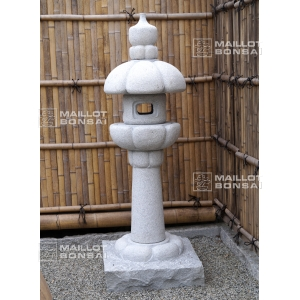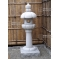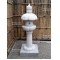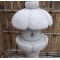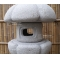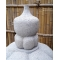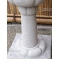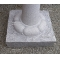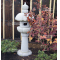ref. : 9524

720,00

voluminous/heavy item extra shipping of 55,00

Available quantity : 2Order

###### Description

Height 120 cm, top section 40*40 cm, weight 180 kilograms, delivered in 6 elements. This solid granite lantern is a rare model in Europe since it’s a copy of a toro from a garden in Kyoto. The reflecting lotus at the base is joined by a sinuous pole that leads up to a supporting lotus and compartment with framed square windows. A bulb can be added to illuminate it, lighting pathways and entrances. Japanese name :"yoshino gata"

#lantern 5.7 #stone 4.5 #yoshino 3.7 #granite 3.7 #gata 3.4 #lotus 2.5 #compartment 2.1 #illuminate 2 #supporting 2 #reflecting 2

Formule
(( ROUND((CHAR_LENGTH(b.article_nom)-CHAR_LENGTH(REPLACE(b.article_nom, 'lantern', '')))/LENGTH('lantern')) + ROUND((CHAR_LENGTH(b.article_description)-CHAR_LENGTH(REPLACE(b.article_description, 'lantern', '')))/LENGTH('lantern')) ) * 3.7) + (( ROUND((CHAR_LENGTH(b.article_nom)-CHAR_LENGTH(REPLACE(b.article_nom, 'yoshino', '')))/LENGTH('yoshino')) + ROUND((CHAR_LENGTH(b.article_description)-CHAR_LENGTH(REPLACE(b.article_description, 'yoshino', '')))/LENGTH('yoshino')) ) * 3.7) + (( ROUND((CHAR_LENGTH(b.article_nom)-CHAR_LENGTH(REPLACE(b.article_nom, 'granite', '')))/LENGTH('granite')) + ROUND((CHAR_LENGTH(b.article_description)-CHAR_LENGTH(REPLACE(b.article_description, 'granite', '')))/LENGTH('granite')) ) * 3.7) + (( ROUND((CHAR_LENGTH(b.article_nom)-CHAR_LENGTH(REPLACE(b.article_nom, 'gata', '')))/LENGTH('gata')) + ROUND((CHAR_LENGTH(b.article_description)-CHAR_LENGTH(REPLACE(b.article_description, 'gata', '')))/LENGTH('gata')) ) * 3.4) + (( ROUND((CHAR_LENGTH(b.article_nom)-CHAR_LENGTH(REPLACE(b.article_nom, 'stone', '')))/LENGTH('stone')) + ROUND((CHAR_LENGTH(b.article_description)-CHAR_LENGTH(REPLACE(b.article_description, 'stone', '')))/LENGTH('stone')) ) * 2.5) + (( ROUND((CHAR_LENGTH(b.article_nom)-CHAR_LENGTH(REPLACE(b.article_nom, 'lotus', '')))/LENGTH('lotus')) + ROUND((CHAR_LENGTH(b.article_description)-CHAR_LENGTH(REPLACE(b.article_description, 'lotus', '')))/LENGTH('lotus')) ) * 2.5) + (( ROUND((CHAR_LENGTH(b.article_nom)-CHAR_LENGTH(REPLACE(b.article_nom, 'compartment', '')))/LENGTH('compartment')) + ROUND((CHAR_LENGTH(b.article_description)-CHAR_LENGTH(REPLACE(b.article_description, 'compartment', '')))/LENGTH('compartment')) ) * 2.1) + (( ROUND((CHAR_LENGTH(b.article_nom)-CHAR_LENGTH(REPLACE(b.article_nom, 'illuminate', '')))/LENGTH('illuminate')) + ROUND((CHAR_LENGTH(b.article_description)-CHAR_LENGTH(REPLACE(b.article_description, 'illuminate', '')))/LENGTH('illuminate')) ) * 2) + (( ROUND((CHAR_LENGTH(b.article_nom)-CHAR_LENGTH(REPLACE(b.article_nom, 'supporting', '')))/LENGTH('supporting')) + ROUND((CHAR_LENGTH(b.article_description)-CHAR_LENGTH(REPLACE(b.article_description, 'supporting', '')))/LENGTH('supporting')) ) * 2) + (( ROUND((CHAR_LENGTH(b.article_nom)-CHAR_LENGTH(REPLACE(b.article_nom, 'reflecting', '')))/LENGTH('reflecting')) + ROUND((CHAR_LENGTH(b.article_description)-CHAR_LENGTH(REPLACE(b.article_description, 'reflecting', '')))/LENGTH('reflecting')) ) * 2)

## Secure payment## Delivery

Our logistic partners :04 74 55 23 48
Pépinière MAILLOT-BONSAÏ
Le Bois Frazy
01990 RELEVANT - FRANCE
on appointment